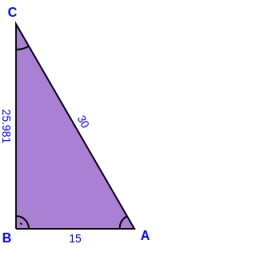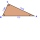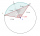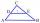# Right angle

In a right triangle ABC with a right angle at the apex C, we know the side length AB = 24 cm and the angle at the vertex B = 71°. Calculate the length of the legs of the triangle.

a =  7.8136 cm
b =  22.6924 cm

### Step-by-step explanation:

Try calculation via our triangle calculator.Did you find an error or inaccuracy? Feel free to write us. Thank you!Tips to related online calculators
Pythagorean theorem is the base for the right triangle calculator.

#### You need to know the following knowledge to solve this word math problem:

We encourage you to watch this tutorial video on this math problem:

## Related math problems and questions:

• RectangularRectangular triangle KLM with right angle at vertex L, angle beta at vertex K and angle alpha at vertex M. Angle at vertex M = 65°, side l = 17.5 cm. Use Pythagorean theorems and trigonometric functions to calculate the lengths of all sides and the angle
• Right triangle trigonometricsCalculate the size of the remaining sides and angles of a right triangle ABC if it is given: b = 10 cm; c = 20 cm; angle alpha = 60° and the angle beta = 30° (use the Pythagorean theorem and functions sine, cosine, tangent, cotangent)
• Outer anglesThe outer angle of the triangle ABC at the A vertex is 71°40 ' outer angle at the vertex B is 136°50'. What size has the inner triangle angle at the vertex C?
• The right triangleIn the right triangle ABC with a right angle at C, we know the side lengths AC = 9 cm and BC = 7 cm. Calculate the length of the remaining side of the triangle and the size of all angles.
• Length of the chordCalculate the length of the chord in a circle with a radius of 25 cm with a central angle of 26°.
• Diagonal BDFind the length of the diagonal BD in a rectangular trapezoid ABCD with a right angle at vertex A when/AD / = 8,1 cm and the angle DBA is 42°
• Diamond and anglesFind the area of a diamond with a side of 5 cm if you know that the internal angles in the diamond are 60° and 120°.
• Space diagonal anglesCalculate the angle between the body diagonal and the side edge c of the block with dimensions: a = 28cm, b = 45cm and c = 73cm. Then, find the angle between the body diagonal and the plane of the base ABCD.
• Right triangleCalculate the length of the remaining two sides and the angles in the rectangular triangle ABC if a = 10 cm, angle alpha = 18°40'.
• MedianIn triangle ABC is given side a=10 cm and median ta= 13 cm and angle gamma 90°. Calculate length of the median tb.
• A trapezoidA trapezoid with a base length of a = 36.6 cm, with angles α = 60°, β = 48° and the height of the trapezoid is 20 cm. Calculate the lengths of the other sides of the trapezoid.
• Pyramid 8Calculate the volume and the surface area of a regular quadrangular pyramid with the base side 9 cm and side wall with the base has an angle 75°.
• Centre of the hypotenuseFor the interior angles of the triangle ABC, alpha beta and gamma are in a ratio of 1: 2: 3. The longest side of the AB triangle is 30 cm long. Calculate the perimeter of the triangle CBS if S is the center of the side AB.
• ParallelogramFind the parallelogram's perimeter, where base a = 8 cm, height v = 3 cm, and angle alpha = 35° is the magnitude of the angle at vertex A.
• Segment in a triangleIn a triangle ABC with the side/AB/ = 24 cm is constructed middle segment/DE/ = 18 cm parallel to the side AB at a distance 1 cm from AB. Calculate the height of the triangle ABC to side AB.
• Parallelogram ABCDWe have the parallelogram ABCD, where AB is 6.2 cm BC is 5.4 cm AC is 4.8 cm calculate the height on the AB side and the angle DAB
• Right triangleIt is given a right triangle angle alpha of 90 degrees beta angle of 55 degrees c = 10 cm use Pythagorean theorem to calculate sides a and b